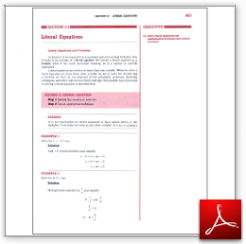Algebra Math Book - Introductory Algebra Thank you for your continual support.

 The power of mathematics rests in the logic of thinking.ID: Sec6-9
Description: Literal Equations and Formulas
Price: 1.99

# Algebra Math book - Introductory Algebra - Chapter 6 - Section 9 - Literal Equations

## Section 6.9 - Objectives

14.  Solve literal equations and mathematical formulas that contain fractions.

Navigate to
Previous Section or Next Chapter
or Chapter 6 Details or Other Chapters

This section of my algebra math book, Introductory Algebra, also includes in the download:

• Cover Sheet